# WYE to DELTA and DELTA to WYE CONVERSION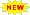Click or Tap the Example circuits below to invoke TINACloud and select the Interactive DC mode to Analyze them Online.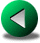SERIES-PARALLEL CONNECTED RESISTORSVOLTAGE DIVISION

In many circuits, resistors are neither in series nor in parallel, so the rules for series or parallel circuits described in previous chapters cannot be applied. For these circuits, it may be necessary to convert from one circuit form to another to simplify the solution. Two typical circuit configurations that often have these difficulties are the wye (Y) and delta ( D ) circuits. They are also referred to as tee (T) and pi ( P ) circuits, respectively.

Delta and wye circuits: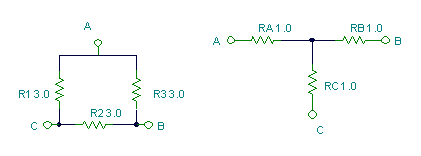And the equations for converting from delta to wye: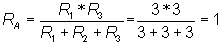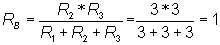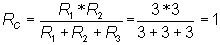The equations can be presented in an alternate form based on the total resistance (Rd) of R1, R2, and R3 (as though they were placed in series):

Rd = R1+R2+R3

and:

RA = (R1*R3) / Rd

RB = (R2*R3) / Rd

RC = (R1*R2) / Rd

Wye and delta circuits: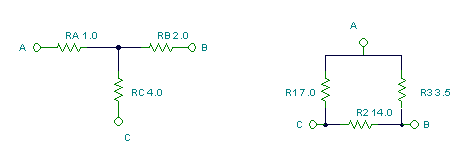And the equations for converting from wye to delta: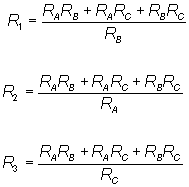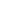An alternate set of equations can be derived based on the total conductance (Gy) of RA, RB, and RC (as though they were placed in parallel):

Gy = 1/RA+1/RB+1/RC

and:

R1 = RB*RC*Gy

R2 = RA*RC*Gy

R3 = RA*RB*Gy

The first example uses the delta to wye conversion to solve the well-known Wheatstone bridge.

Example 1

Find the equivalent resistance of the circuit !

Click/tap the circuit above to analyze on-line or click this link to Save under Windows

Notice that the resistors are connected neither in series nor in parallel, so we can't use the rules for series or parallel connected resistors

Let's choose the delta of R1,R2 and R4:and convert it to a star circuit of RA, RB, RC.

Click/tap the circuit above to analyze on-line or click this link to Save under Windows

Using the formulas for the conversion: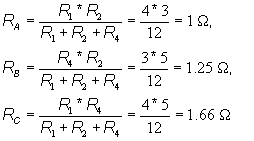After this transformation, the circuit contains only resistors connected in series and parallel. Using the series and parallel resistance rules, the total resistance is: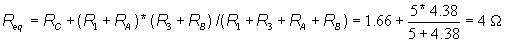Now let's use TINA's Interpreter to solve the same problem, but this time we will use wye to delta conversion. First, we convert the wye circuit consisting of R1, R1, and R2. Since this wye circuit has two arms of the same resistance, R1, we have only two equations to solve. The resulting delta circuit will have three resistors, R11, R12, and R12.

: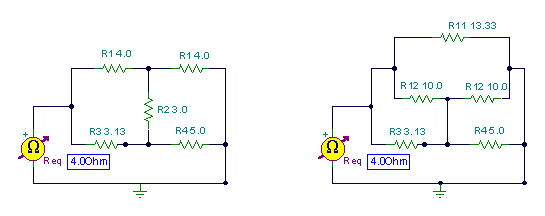Solution by TINA's Interpreter

Gy:=1/R1+1/R1+1/R2;

Gy=[833.3333m]

R11:=R1*R1*Gy;

R12:=R1*R2*Gy;

Using TINA's function for parallel impedances, Replus:

Req:=Replus(R11,(Replus(R12,R3)+Replus(R12,R4)));

Req=[4.00]

Example 2

Find the resistance shown by the meter !

Click/tap the circuit above to analyze on-line or click this link to Save under Windows

Let's convert the R1, R2, R3 wye network to a delta network. This conversion is the best choice for simplifying this network.

Solution by TINA's Interpreter

First, we do the wye to delta conversion, then we notice the instances of paralleled resistors in the simplified circuit.

{wye to delta conversion for R1, R2, R3 }

Gy:=1/R1+1/R2+1/R3;

Gy=[95m]

RA:=R1*R2*Gy;

RB:=R1*R3*Gy;

RC:=R2*R3*Gy;

Req:=Replus(Replus(R6,RB),(Replus(R4,RA)+Replus(R5,RC)));

RA=

RB=

RC=

Req=

Example 3

Find the equivalent resistance shown by the meter !

Click/tap the circuit above to analyze on-line or click this link to Save under Windows

This problem offers many possibilities for conversion. It is important to find which wye or delta conversion makes the shortest solution. Some work better then others while some may not work at all.

In this case, let's start by using delta to wye conversion of R1, R2 and R5. We will next have to use wye to delta conversion. Study the Interpreter equations below carefully

Click/tap the circuit above to analyze on-line or click this link to Save under Windows

for RAT, RB, RCT: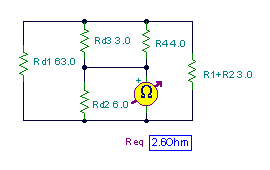Solution by TINA's Interpreter

Rd:=R1+R2+R5;

Rd=

RC:=R1*R5/Rd;

RB:=R1*R2/Rd;

RA:=R2*R5/Rd;

{Let be (R1+R3+RA)=RAT=5.25 ohm; (R2+RC) = RCT = 2.625 ohm.

Using a wye to delta conversion for RAT, RB, RCT !}

RAT:=R1+R3+RA;

RCT:=R2+RC;

Gy:=1/RAT+1/RB+1/RCT;

Rd2:=RB*RAT*Gy;

Rd3:=RB*RCT*Gy;

Rd1:=RCT*RAT*Gy;

Req:=Replus(Rd2,(Replus(R4,Rd3)+Replus(Rd1,(R1+R2))));

Req=[2.5967]